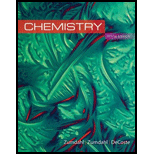# The activation energy for the reaction NO 2 ( g ) + CO ( g ) → NO ( g ) + CO 2 ( g ) is 125 kJ/mol, and ∆ E for the reaction is −216 kJ/mol. What is the activation energy for the reverse reaction [NO( g ) + CO 2 ( g ) →+ NO 2 ( g ) + CO( g )]?### Chemistry

10th Edition
Steven S. Zumdahl + 2 others
Publisher: Cengage Learning
ISBN: 9781305957404

#### Solutions

Chapter
Section### Chemistry

10th Edition
Steven S. Zumdahl + 2 others
Publisher: Cengage Learning
ISBN: 9781305957404
Chapter 12, Problem 69E
Textbook Problem
41 views

## The activation energy for the reaction NO 2 ( g )   +  CO ( g )   →  NO ( g )   +  CO 2 ( g ) is 125 kJ/mol, and ∆E for the reaction is −216 kJ/mol. What is the activation energy for the reverse reaction [NO(g) + CO2(g) →+ NO2(g) + CO(g)]?

Interpretation Introduction

Interpretation: The activation energy and ΔE for a reaction is given. By using these values, the activation energy for the given reverse reaction is to be calculated.

Concept introduction: A certain threshold energy which is necessary for the reaction to occur is called activation energy.

The relationship between the rate constant and activation energy is given by the Arrhenius equation,

k=AeEaRT

The change in energy (ΔE) is the energy difference between reactant and product. The value of ΔE is either positive or negative.

To determine: The activation energy for the given reverse reaction.

### Explanation of Solution

Given

The activation energy of given forward reaction is 125kJ/mol .

The change in energy ΔE is 216kJ/mol .

The given reverse reaction is,

NO(g)+CO2(g)NO2(g)+CO(g)

Formula

The change in energy is calculated by using the formula,

ΔE=Ea(forward)Ea(backward)

Where,

• ΔE is change in energy.
• Ea(forward) is activation energy of forward reaction

### Still sussing out bartleby?

Check out a sample textbook solution.

See a sample solution

#### The Solution to Your Study Problems

Bartleby provides explanations to thousands of textbook problems written by our experts, many with advanced degrees!

Get Started

Find more solutions based on key concepts
The theory that vitamin C prevents or cures colds is well supported by research. T F

Nutrition: Concepts and Controversies - Standalone book (MindTap Course List)

1-24 Solve: (9.52104)(2.77105)(1.39107)(5.83102)

Introduction to General, Organic and Biochemistry

Whats a suspension feeder?

Oceanography: An Invitation To Marine Science, Loose-leaf Versin

You are riding on a Ferris wheel that is rotating with constant speed. The car in which you are riding always m...

Physics for Scientists and Engineers, Technology Update (No access codes included)